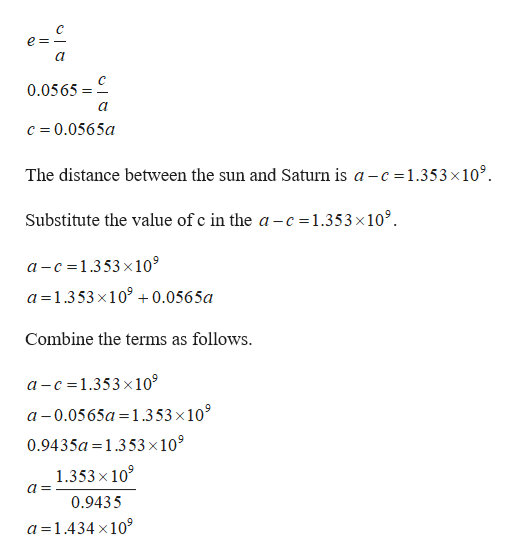# The planet Saturn orbits the Sun in an elliptical path with the Sun at one focus. The eccentricity of the orbit is 0.0565 and the distance between the Sun and Saturn at perihelion (the closest point) is 1.353 × 109km. Determine the distance at aphelion (the farthest point). Round to the nearest million kilometers.

Question
46 views

The planet Saturn orbits the Sun in an elliptical path with the Sun at one focus. The eccentricity of the orbit is 0.0565 and the distance between the Sun and Saturn at perihelion (the closest point) is 1.353 × 109km. Determine the distance at aphelion (the farthest point). Round to the nearest million kilometers.

check_circle

Step 1

It is given that the eccentricity of the orbit is 0.0565 and the distance between the sun and Saturn at perih...help_outlineImage Transcriptionclose0.0565 c = 0.0565a The distance between the sun and Saturn is a - =1.353×10°. Substitute the value of c in the a-c =1.353×10°. a-c =1.353x10° a =1.353 x10° +0.0565a Combine the terms as follows. a - c =1.353×10° a -0.0565a =1.353×10° %3D 0.9435a =1.353 ×10° 1.353 x 10° 0.9435 a =1.434 x10° fullscreen

### Want to see the full answer?

See Solution

#### Want to see this answer and more?

Solutions are written by subject experts who are available 24/7. Questions are typically answered within 1 hour.*

See Solution
*Response times may vary by subject and question.
Tagged in

### Algebra